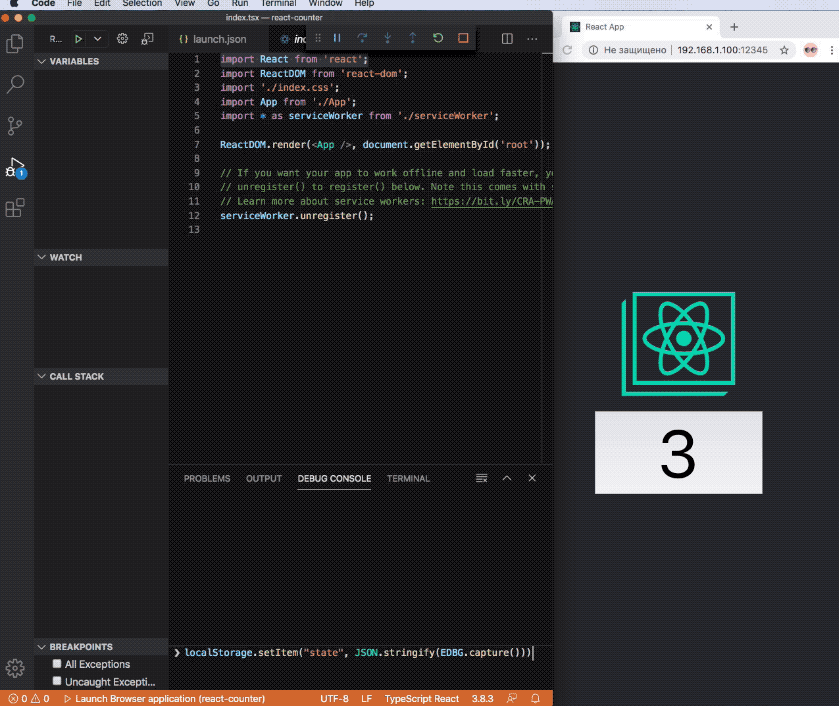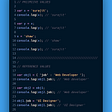# Trailing commas in array

`let a = [, , ,];a.length; // -> 3a.toString(); // -> ',,'`

# Be careful while comparing the arrays.

`[] == ''   // -> true[] == 0    // -> true[''] == '' // -> true == 0   // -> true == ''  // -> false[''] == 0  // -> true[null] == ''      // -> true[null] == 0       // -> true[undefined] == '' // -> true[undefined] == 0  // -> true`
1. If x is null and y is undefined, return true.
2. If x is undefined and y is null, return true.
3. If Type(x) is Number and Type(y) is String, return the result of the comparison x == ! ToNumber(y).
4. If Type(x) is String and Type(y) is Number, return the result of the comparison ! ToNumber(x) == y.
5. If Type(x) is String and Type(y) is BigInt, return the result of the comparison y == x.
6. If Type(x) is Boolean, return the result of the comparison ! ToNumber(x) == y.
7. If Type(y) is Boolean, return the result of the comparison x == ! ToNumber(y).
8. If Type(x) is either String, Number, BigInt, or Symbol and Type(y) is Object, return the result of the comparison x == ToPrimitive(y).
9. If Type(x) is Object and Type(y) is either String, Number, BigInt, or Symbol, return the result of the comparison ToPrimitive(x) == y.

# Directly add value at “Nth” place

`let arr = []arr = 'value'`
`let arr = []arr = 'value'arr.length  // -> 100arr      // -> undefinedas same aslet a;console.log(a)  // -> undefined`

# Math with “`true”` and “`false"`

`true + true;                          // -> 2(true + true) * (true + true) - true; // -> 3`

# Comparison of three numbers

`1 < 2 < 3; // -> true3 > 2 > 1; // -> false`
`expression : 1 < 2 < 3;step 1 : (1 < 2) < 3step 2 : true < 3step 3 : 1 < 3        // true is coerce as 1answer : true`
`expression : 3 > 2 > 1;step 1 : (3 > 2) > 1step 2 : true > 1step 3 : 1 > 1     // true is coerce as 1answer : false`

--

--

--

## More from Pratik Das

Software Engineer

Love podcasts or audiobooks? Learn on the go with our new app.

## Extension types in TypeScript## Promise.all, Promise.race, and the new ES2020 Promise.allSettled## 3 Steps to the Clean Code: NodeJS + NestJS project tips.## Arrow Functions vs Regular Functions: JavaScript Basics## Productivity-boosting JavaScript debugger## JavaScript core concepts — part 1## m-n Associations in Sequelize## Pratik Das

Software Engineer

## How do JavaScript arrow functions work and when should you use them?## Use normalize() to duel with non-English string in javascript## Reference VS. Primitive Values